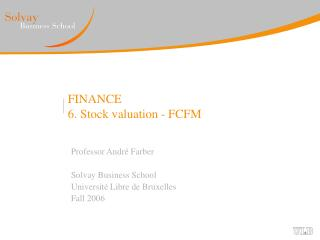Download PresentationFINANCE 6. Stock valuation - FCFM

# FINANCE 6. Stock valuation - FCFM

Download Presentation## FINANCE 6. Stock valuation - FCFM

- - - - - - - - - - - - - - - - - - - - - - - - - - - E N D - - - - - - - - - - - - - - - - - - - - - - - - - - -
##### Presentation Transcript

1. FINANCE6. Stock valuation - FCFM Professor André Farber Solvay Business School Université Libre de Bruxelles Fall 2006

2. Stock Valuation • Objectives for this session : • Review the dividend discount model (DDM) • Understand the sources of dividend growth • Analyse growth opportunities • Examine why Price-Earnings ratios vary across firms • Introduce free cash flow model (FCFM) MBA 2006 FCFM

3. Dividend Discount Model • General formula: • r is the required return • Constant growth model MBA 2006 FCFM

4. A formula for g • Dividend are paid out of earnings: • Dividend = Earnings × Payout ratio • Payout ratios of dividend paying companies tend to be stable. • Growth rate of dividend g = Growth rate of earnings • Earnings increase because companies invest. • Net investment = Retained earnings • Growth rate of earnings is a function of: • Retention ratio = 1 – Payout ratio • Return on Retained Earnings g = (Return on Retained Earnings) × (Retention Ratio) MBA 2006 FCFM

5. NPVGO • Additional value if the firm retains earnings in order to fund new projects • where PV(NPVt) is the present value at time 0 of the net present value (calculated at time t) of a future investment at time t MBA 2006 FCFM

6. What Do Price-Earnings Ratios mean? • Definition: P/E = Stock price / Earnings per share • Why do P/E vary across firms? • As: P0 = EPS/r + NPVGO  • Three factors explain P/E ratios: • Accounting methods: • Accounting conventions vary across countries • The expected return on shareholders’equity • Risky companies should have low P/E • Growth opportunities MBA 2006 FCFM

7. Beyond DDM: The Free Cash Flow Model • Consider an all equity firm. • If the company: • Does not use external financing (not stock issue, # shares constant) • Does not accumulate cash (no change in cash) • Then, from the cash flow statement: • Free cash flow = Dividend • CF from operation – Investment = Dividend • Company financially constrained by CF from operation • If external financing is a possibility: • Free cash flow = Dividend – Stock Issue • Market value of company = PV(Free Cash Flows) MBA 2006 FCFM

8. FCFM: example Euro m Current situation # shares: 100m Market value of company (r = 10%) V0 = 100/0.10 = €1,000mPrice per share P0 = €1,000m / 100m = €10 Project MBA 2006 FCFM

9. Free Cash Flow Calculation MBA 2006 FCFM

10. Self financing – DIV = FCF, no stock issue Market value of equity with project: (As the number of shares is constant, discounting free cash flows or total dividends leads to the same result) NPV = increase in the value of equity due to project NPV = 1,694 – 1,000 = 694 MBA 2006 FCFM

11. Outside financing : Dividend = Net Income, SI = Div. – FCF Market value of equity with project: (Discount free cash flow, not total dividends) Same value as before! MBA 2006 FCFM

12. Why not discount total dividends? Because part of future total dividends will be paid to new shareholders. They should not be taken into account to value the shares of current shareholders. To see this, let us decompose each year the value of all shares between old shares (those outstanding one year before) and new shares (those just issued) MBA 2006 FCFM

13. The price per share is obtained by dividing the market value of old share by the number of old shares: Year 1: Number of old shares = 100 P1 = 1,764 / 100 = 17.64 The number of shares to issue is obtained by dividing the total stock issue by the number of shares: Year 1: Number of new shares issued = 100 / 17.74 = 5.67 Similar calculations for year 2 lead to: Number of old shares = 105.67 Price per share P2 = 1,900 / 105.67 = 17.98 Number of new share issued = 100 / 17.98 = 5.56 MBA 2006 FCFM

14. From DDM to FCFM: formulas • Consider an all equity firm • Value of one share: P0 = (div1 + P1)/(1+r) • Market value of company = value of all shares • V0 = n0P0 = (n0div1 + n0P1)/(1+r) • n0 div1 = total dividend DIV1 paid by the company in year 1 • n0P1 = Value of “old shares” • New shares might be issued (or bought back) in year 1 • V1 = n1P1 = n0P1 + (n1-n0)P1 • Statement of cash flow (no debt, cash constant): • FCF1 = DIV1 – (n1-n0)P1 →DIV1 + n0P1 = FCF1 + V1 • Conclusion: • V0 = (FCF1 +V1) /(1+r) MBA 2006 FCFM